# R<1: Time to lift the lockdown?

Sri Lanka reported its first 350 cases over a period of 44 days. However, it took a mere 10 days to report the last 350! This has naturally led to serious concerns on the viability of relaxing the lockdown measures in the near future.

We intend to present a simple yet powerful approach, well-grounded in scientific methodology, that can be used to devise a suppress-lift strategy. This approach is successfully implemented in many countries to devise the suppress-lift strategy and we hope Sri Lanka too will adopt a similar approach.

This analysis has been conducted in collaboration with Sankha Muthu Poruthotage, Ishanga Udatiyawala, Vanodya Perera and Ishan Buddhika.

General Approach

There are three key steps in the process of predicting the spread of the virus.

1. Adjusting the reported cases for reporting lag, which is called now-casting

2. Calculating the applicable reproduction number

3. Simulating the future spread of the virus

Since we have already discussed now-casting in this post, let us not elaborate on the same. For completeness though, the following figure compares the actual infections vs reported cases on a daily basis.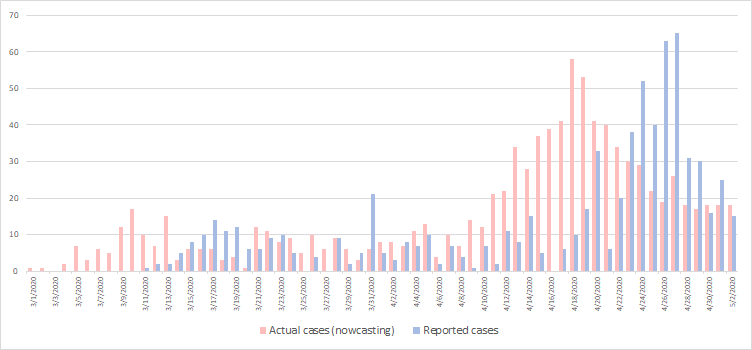Comparison between daily nowcasted infection cases and daily reported cases (Results of a statistical method called nowcasting that adjusts for reporting and detection delays)

Calculating the Applicable Reproduction Number

The basic reproduction number R0 is the average number of secondary infections caused by one primary infection. For Sri Lanka, this number has been estimated to be around1.4–2.5 (source). If R0 is below one, the epidemic eventually peters out. Above one, it will grow, possibly exponentially.

However, in order to calculate this number empirically, perfect contact tracings need to be carried out. This may not be always possible given the practical limitations. Hence, we are presenting a statistical modelling approach to calculate the reproduction number in real-time.

Reproduction number can vary from place to place. It will also vary with time — depending on the level of social interactions prevailing in a society. However, if the effective reproduction number (Rt) can be estimated in real-time, the disease spread can be forecasted with high degree of accuracy.

Prior to that, the generation lag distribution needs to be determined. Generation lag can be defined as the time between symptom onset in a primary case and a secondary case. Based on previous research, we assumed a gamma distribution with a mean of 5.8 days and a standard deviation of 2.6 days as the generation lag, in order to obtain real-time reproduction numbers.

Once the generation lag distribution and daily infected cases are identified, we have used the time dependent method for Rt calculation, implemented in this article.

The following figure represents the effective reproduction rate (Rt) for Covid 19 in Sri Lanka.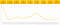Real-time reproduction number (Rt) obtained using nowcast infections

Simulating the Spread of COVID-19 Virus

Our objective of finding the actual cases and Rt was to simulate the futuristic behaviour for different scenarios based on the SIR (Susceptible — Infected — Removed) model. This is a compartment model which explains how individuals in a community move through 3 different compartments in an outbreak situation.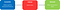Compartments of SIR model

Initially, the entire population would fall under Susceptible compartment and then would move through Infected and Removed compartments based on Rt and other transmission and clinical dynamics.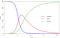A simulated sample SIR model with an initial population of 10,000

We found this tool that gives us simulations based on SIR model which uses transmission and clinical dynamics for a specified initial date as inputs and simulate the behaviour based on changes to Rt after a specific intervention date.

For the purpose of this analysis, 2nd May 2020 was considered as the initial point (Day 0) with a reproduction number of 0.83. Active cases as of Day 0 stood at 768 (obtained by subtracting the recovered count (182) and death count (7) from the nowcast cumulative (957)). Below are the other transmission and clinical dynamics considered for the simulation.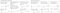Transmission and clinical dynamics used for simulation

Following simulation illustrates that the total number of active infections will come down quite drastically over the next 2 weeks! However, this is strictly under the assumption that the current Rt value (=0.83) is prevailing indefinitely into the future.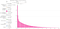Active cases assuming Rt=0.83 to be continued

Devising a Suppress-Lift Strategy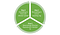3-step cycle for devising a suppress-lift strategy

When some of the lockdown measures are relaxed, it is possible that Rt may start moving upwards once again. This will lead to more active infections and burden the socio-economic wellbeing of the nation. Therefore, it is absolutely necessary that the 3-step process we have discussed here is continuously updated and monitored as a cycle.

Data Scientist at Linear Squared

## More from Ayesha Kasturiarachchi

Data Scientist at Linear Squared